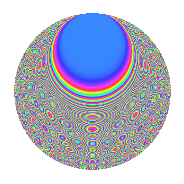# Properties

 Label 1350.2.zLevel 1350 Weight 2 Character orbit z Rep. character $$\chi_{1350}(19,\cdot)$$ Character field $$\Q(\zeta_{30})$$ Dimension 240 Sturm bound 540

# Related objects

## Defining parameters

 Level: $$N$$ = $$1350 = 2 \cdot 3^{3} \cdot 5^{2}$$ Weight: $$k$$ = $$2$$ Character orbit: $$[\chi]$$ = 1350.z (of order $$30$$ and degree $$8$$) Character conductor: $$\operatorname{cond}(\chi)$$ = $$225$$ Character field: $$\Q(\zeta_{30})$$ Sturm bound: $$540$$

## Dimensions

The following table gives the dimensions of various subspaces of $$M_{2}(1350, [\chi])$$.

Total New Old
Modular forms 2256 240 2016
Cusp forms 2064 240 1824
Eisenstein series 192 0 192

## Trace form

 $$240q - 30q^{4} + 8q^{5} + O(q^{10})$$ $$240q - 30q^{4} + 8q^{5} + 4q^{11} - 8q^{14} + 30q^{16} + 2q^{20} + 24q^{25} + 96q^{26} - 12q^{29} + 12q^{31} + 32q^{35} + 16q^{41} + 8q^{44} + 50q^{47} + 120q^{49} + 4q^{50} + 24q^{55} + 8q^{56} - 18q^{59} + 60q^{62} + 60q^{64} + 64q^{65} - 30q^{67} + 24q^{70} - 76q^{71} + 80q^{74} - 80q^{77} + 12q^{79} + 4q^{80} + 140q^{83} + 12q^{85} + 20q^{86} + 28q^{89} + 40q^{92} - 36q^{95} + O(q^{100})$$

## Decomposition of $$S_{2}^{\mathrm{new}}(1350, [\chi])$$ into newform subspaces

The newforms in this space have not yet been added to the LMFDB.

## Decomposition of $$S_{2}^{\mathrm{old}}(1350, [\chi])$$ into lower level spaces

$$S_{2}^{\mathrm{old}}(1350, [\chi]) \cong$$ $$S_{2}^{\mathrm{new}}(225, [\chi])$$$$^{\oplus 4}$$$$\oplus$$$$S_{2}^{\mathrm{new}}(450, [\chi])$$$$^{\oplus 2}$$$$\oplus$$$$S_{2}^{\mathrm{new}}(675, [\chi])$$$$^{\oplus 2}$$

## Hecke Characteristic Polynomials

There are no characteristic polynomials of Hecke operators in the database